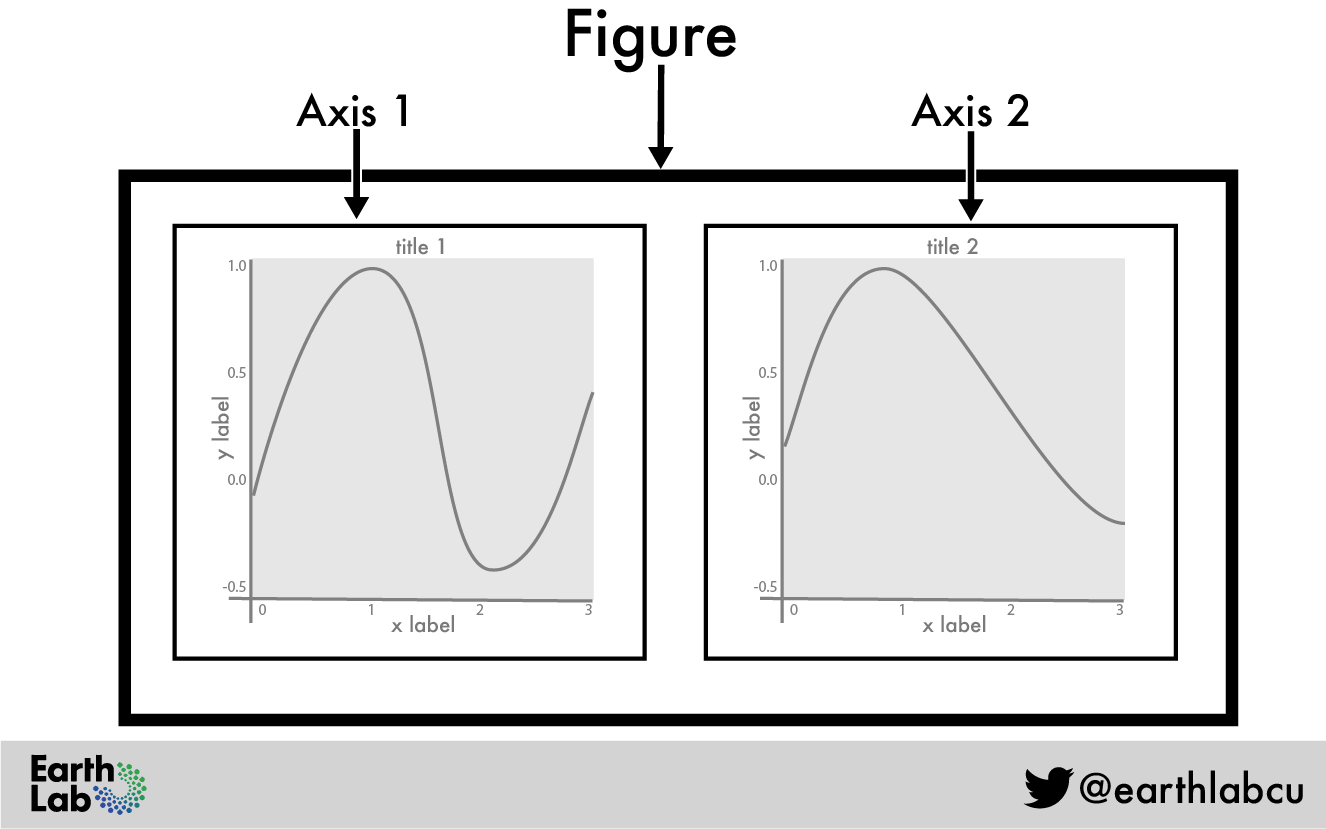# 3pyplot

Matplotlib is probably the most common Python package used for plotting. It is both great and horrible:

• Great: you’ll have absolutely full control of everything you want to plot. The sky is the limit.
• Horrible: you’ll cry as you do it, because there is so much to know, and it is not the most friendly plotting package.

For our needs, matplotlib will be just fine. Actually, we will be using a submodule called Pyplot, and your fingers will learn to type the following line without thinking at the top of every project you start:

``import matplotlib.pyplot as plt``

As for Pandas, you will learn by example, and in my opinion the commands are usually self explanatory. Pyplot is object oriented, so you will usually manipulate the axes object like this.

``````import matplotlib.pyplot as plt

x = [1, 2, 3, 4, 5]
y = [1, 4, 2, 0, 3]

# Figure with two plots
fig, (ax1, ax2) = plt.subplots(1, 2, figsize = (8, 6))
# plot on the left
ax1.plot(x, y, color="tab:blue")
ax1.plot(x, y[::-1], color="tab:orange")
ax1.set(xlabel="date",
ylabel="something",
title="left panel")
# plot on the right
ax2.plot(x, y[::-1])
ax2.set(xlabel="date",
ylabel="something else",
title="right panel")``````
``````[Text(0.5, 0, 'date'),
Text(0, 0.5, 'something else'),
Text(0.5, 1.0, 'right panel')]``````For the very beginners, you need to know that “figure” refers to the whole white canvas, and “axes” means the rectangle inside which something will be plotted:If you are new to all this, I recommend that you go to Earth Lab’s Introduction to Plotting in Python Using Matplotlib, and to Jake VanderPlas’s Python Data Science Handbook